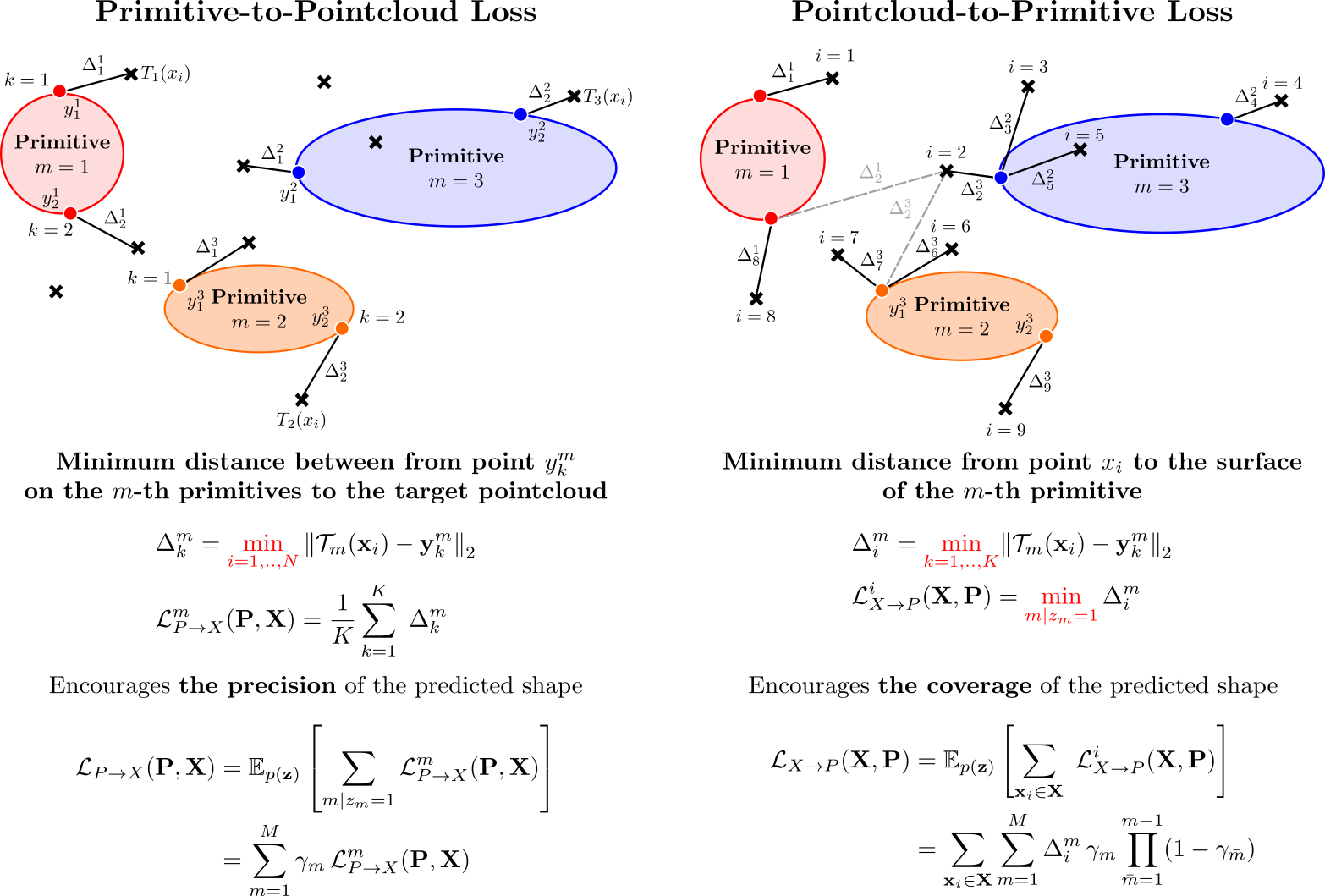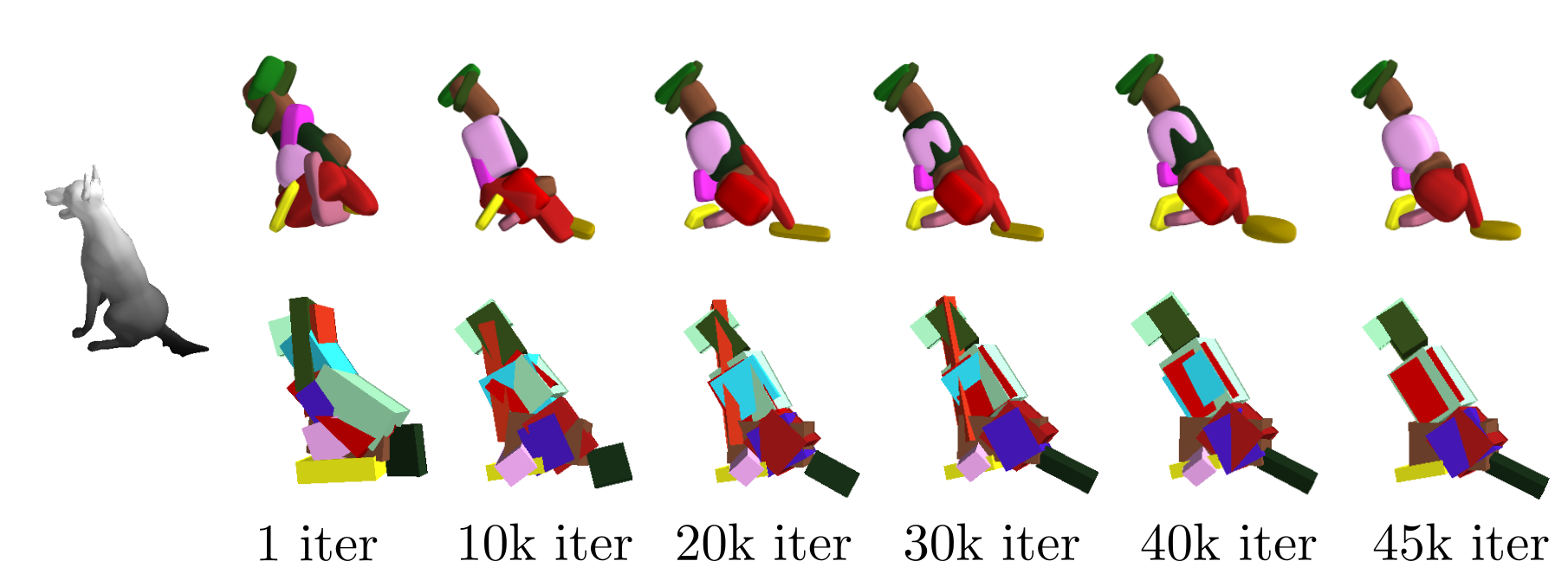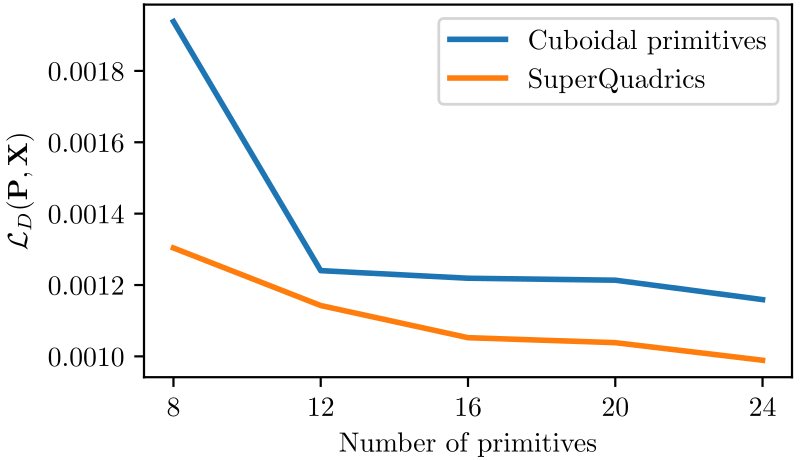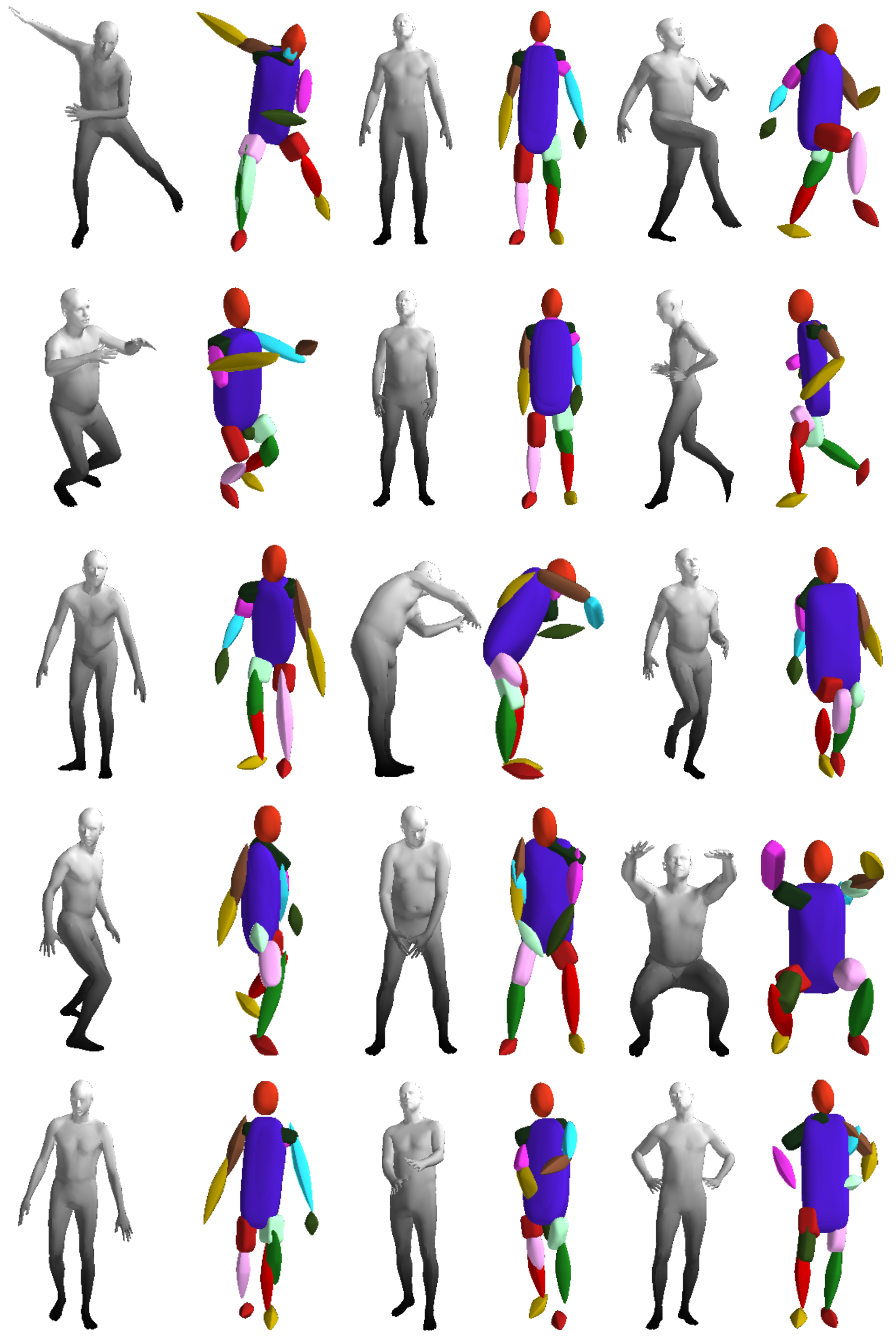# Superquadrics Revisited: Learning 3D Shape Parsing beyond Cuboids

1 Autonomous Vision Group, MPI for Intelligent Systems Tübingen 2 Microsoft
3 University of Tübingen 4 Max Planck ETH Center for Learning Systems
CVPR 2019Superquadrics allow for modeling fine details such as the tails and ears of animals as well as the wings and the body of the airplanes and wheels of the motorbikes which are hard to capture using cuboids
Abstracting complex 3D shapes with parsimonious part-based representations has been a long standing goal in computer vision. This paper presents a learning-based solution to this problem which goes beyond the traditional 3D cuboid representation by exploiting superquadrics as atomic elements. We demonstrate that superquadrics lead to more expressive 3D scene parses while being easier to learn than 3D cuboid representations. Moreover, we provide an analytical solution to the Chamfer loss which avoids the need for computational expensive reinforcement learning or iterative prediction. Our model learns to parse 3D objects into consistent superquadric representations without supervision. Results on various ShapeNet categories as well as the SURREAL human body dataset demonstrate the flexibility of our model in capturing fine details and complex poses that could not have been modelled using cuboids.
Approach Overview
We propose a novel deep neural network that efficiently learns to parse 3D objects into consistent superquadric representations, without any part-level supervision, conditioned on a 3D shape or 2D image as an input. In particular, our network encodes the input image/shape into a low-dimensional primtive representation $$\mathbf{P}=\{(\lambda_m, \gamma_m)\}_{m=1}^M$$, where $$M$$ is an upper bound to the maximum number of primitives. For each primitive our network regresses:
• The primitive parameters $$\lambda_m$$ (2 for the shape, 3 for the size and 6 for the pose).
• An existence probability $$\gamma_m$$ that indicates whether a particular primitive is part of the assembled object.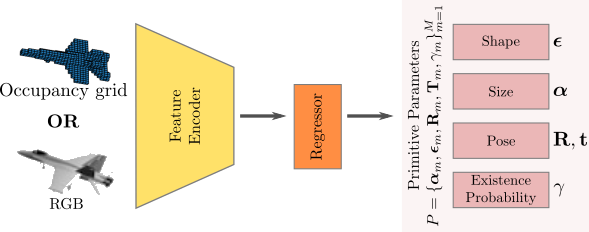We represent the target pointcloud as a set of 3D points $$\mathbf{X}=\{\mathbf{x_i}\}_{i=1}^N$$ and approximate the surface of each primitive $$m$$ using a set of points $$\mathbf{Y}_m = \{\mathbf{y}_k^m\}_{k=1}^K$$ uniformly sampled on the surface of the primitive. To train our network, we measure the discrepancy between the target and the predicted shape. In particular, we formulate our optimization objective as a bi-directional reconstruction loss and incorporate a minimum description length prior, which favors parsimony. In particular, our overall loss is: $$\begin{equation*} \mathcal{L}_{D}(\mathbf{P} , \mathbf{X}) = \underbrace{\mathcal{L}_{P\rightarrow X}(\mathbf{P}, \mathbf{X})}_{ \substack{\text{Primitive-to-Pointcloud}}} + \underbrace{\mathcal{L}_{X\rightarrow P}(\mathbf{X}, \mathbf{P})}_{ \substack{\text{Pointcloud-to-Primitive}}} + \underbrace{\mathcal{L}_{\gamma}(\mathbf{P})}_{ \substack{\text{Parsimony}}} \end{equation*}$$